### 高业务可扩展性的json-diff及可视化工具

MIT
python, React
*
eggachecat · 2021年11月15日 · 1081 次阅读 · 0 条评论

## 哲学

JYCM 选择了另一种方式：使比较值变得容易。

JYCM 允许用户只需要专注于定义不同的逻辑或两个值之间的距离是多少，JYCM 将处理所有其他个比较繁琐的的工作，例如数组项匹配、忽略数组顺序、递归比较和计算相似性。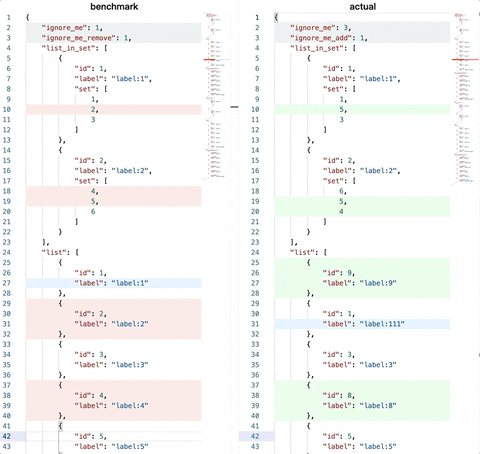## 前言

``````# 基准
left = [
{
"x": 92134,
"y": 42351
}
]
#################################
# 对比
right = [
{
"x": 91111,
"y": 43333
}
]

``````

## JSON-有差吗？

JSON 的基本数据类型：

• 数值：十进制数，不能有前导 0，可以为负数，可以有小数部分。还可以用`e`或者`E`表示指数部分。不能包含非数，如 NaN。不区分整数与浮点数。JavaScript 用双精度浮点数表示所有数值。
• 字符串：以双引号`""`括起来的零个或多个Unicode码位。支持反斜杠开始的转义字符序列
• 布尔值：表示为`true`或者`false`
• 值的有序列表（array）：有序的零个或者多个值。每个值可以为任意类型。序列表使用方括号`[``]`括起来。元素之间用逗号`,`分割。形如：`[value, value]`
• 对象（object）：若干无序的 “键 - 值对”(key-value pairs)，其中键是数值或字符串。建议但不强制要求对象中的键是独一无二的。对象以花括号`{`开始，并以`}`结束。键 - 值对之间使用逗号分隔。键与值之间用冒号`:`分割。
• null 类型：值写为`null`

## object 的有差吗？

object 的 diff 的话是以 key 为唯独，分为三种操作

• 更新
• 新增 key
• 删除 key

``````def diff_dict(left, right):
for k in all_keys:
if k in left and k in right:
# 这个diff跟进类型去选择具体的diff逻辑
diff(left[k], right[k])
continue

if k in left:
# 则执行「新增的逻辑」
pass

if k in right:
# 则执行「删除的逻辑」
pass

``````

## array 有差吗？

array 的 diff 是最特殊的一个，也是最复杂的一个。

``````def diff_array(left, right):
min_len = min([len(left), len(right)]

for i in range(min_len):
diff(left[i], right[i])

if min_len == len(left):
# 则新增啦right[min_len:]这些
pass

if min_len == len(right):
# 则删除了left[min_len:]这些
pass
``````

• 为什么区分有序和无序？
• 以比较「(a,b,c),(c,b,a)」为例，在有序和无序的条件下会有截然不同的结论，因此必须分开对待
• 为什么要区分「精准」和「模糊」匹配，不能直接「模糊匹配」完成嘛？
• 因为模糊匹配本质上是配对问题，找到一个配对的组合，使得这个配对组合的成本最小。
• 以有序的「(a,b,c),(a,z,c)」为例：有可能因为b 和 z的成本过大而导致最终将 (az) 匹配在一起，这个逻辑上并不合理。

• 一个是无法支持「无序」状态下的物理意义
• 另外一个使用这个方法做出来的 diff 的结果并不够完美。
• 以对比「`[a,b,c,d,e]`,`[b,c,d,e,f]`」为例
• index 方法会得到
• 两个数组对应位置的所有元素皆不想等
• 更合理的结论
• 新的数组相比旧的
• 少了第 0 个元素`a`
• 多了最后一个元素`f`

### 有序

• LEFT = (a,b,c,d,e,f,g)
• RIGHT = (t,u,v,c,x,y,e,z)

#### 精准匹配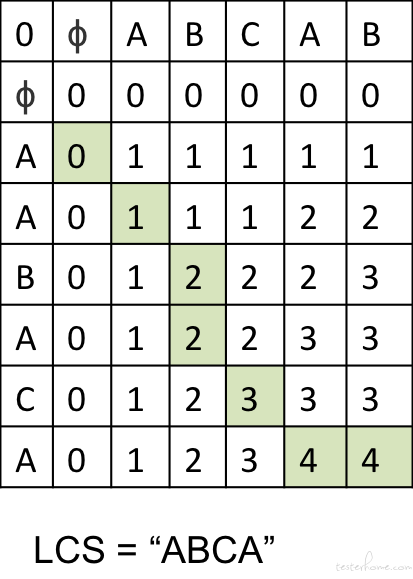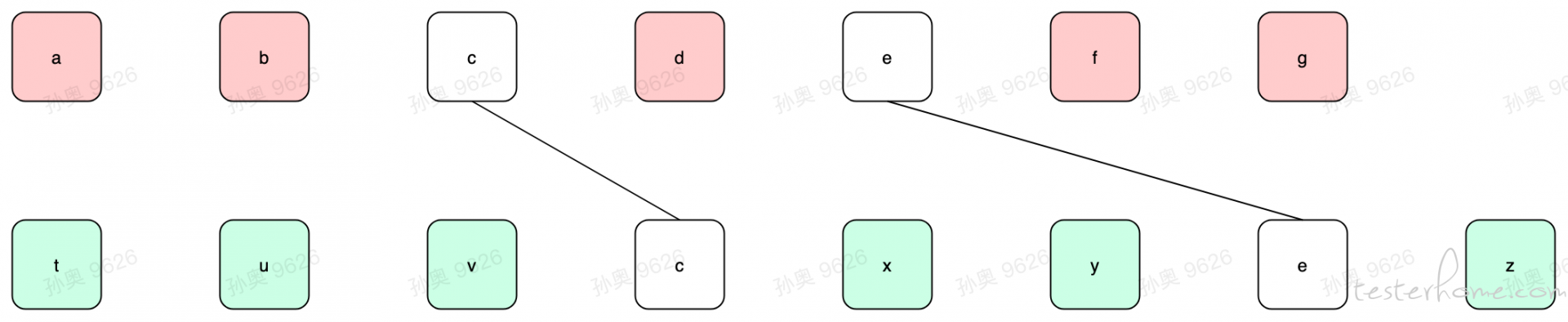#### 模糊匹配

• i.e. 以上图的`LEFT``RIGHT`来说，我们只能用「a,b」和「t,u,v」去做比较，而「a,b」是不能与「x,y」做比较的。
• 原因在于是有序，如果我们把`a``x`匹配了，那么在「`LEFT``a``c`的前面」和「`RIGHT``x``c`后面」就矛盾了。

• 莱文斯坦距离中，可以删除、加入、取代字符串中的任何一个字元，也是较常用的编辑距离定义，常常提到编辑距离时，指的就是莱文斯坦距离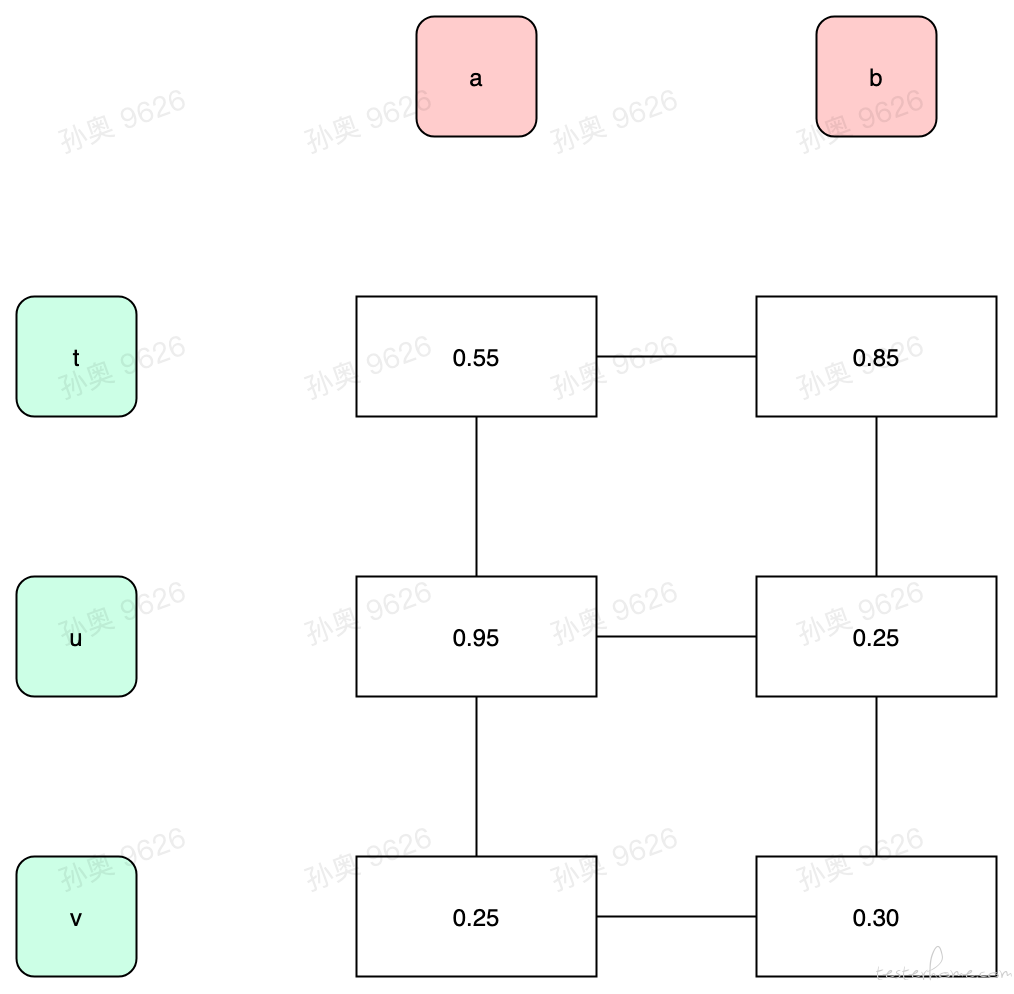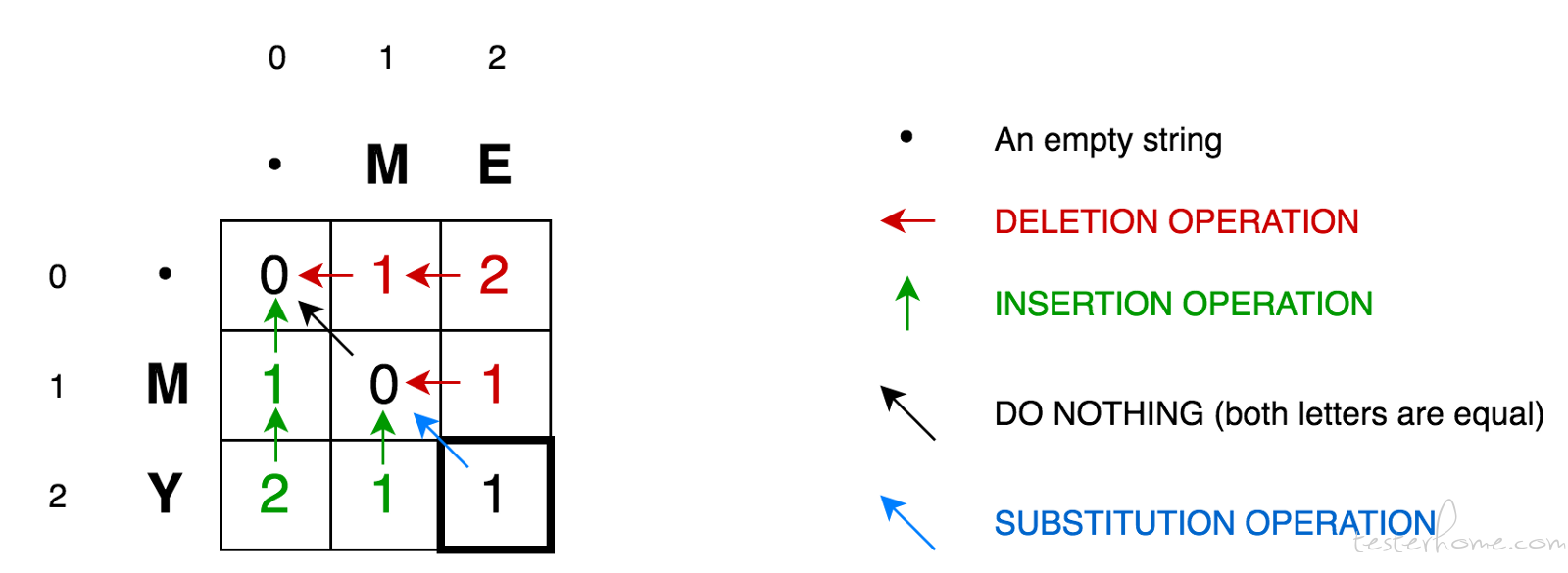### 无序

#### 精准匹配

``````pairs = []
matched = {}

for li in range(len(left)):
for ri in range(len(right)):
if ri in matched:
continue
same = diff(left[li], right[ri])
if same:
pairs.append([left[li], right[ri]])
matched[ri] = True
break
``````

## 递归

``````# 基准
left = {
"id": 1,
"random": 12345，
"set": [
{"id":1, "set": [1,2,3]},
{"id":2, "set": [4,5,6]}
]
}
#################################
# 对比
right = {
"id": 3,
"random": 6784，
"set": [
{"id":2, "set": [6,4,5]},
{"id":1, "set": [3,1,2]},
]
}

``````

## 分数

• 两个 object 的相似度就是所有的 key-value 对的相似度的和
• 两个 array 的相似度就是匹配完元素以后的相似度的和

## 区分「报告阶段」和「比较阶段」

``````# 基准
left = [
{
"id": 1,
"label": "label-1"，
},
{
"id": 2,
"label": "label-2"，
}
]
#################################
# 对比
right = [
{
"id": 0,
"label": "label-0"，
},
{
"id": 1,
"label": "label-1111"，
},
{
"id": 2,
"label": "label-2222"，
}
]
``````

## 用户自定义「相似」

``````# 基准
left = [
{
"x": 92134,
"y": 42351
}
]
#################################
# 对比
right = [
{
"x": 91111,
"y": 43333
}
]

``````

``````def test_operator_custom():
class L2DistanceOperator(BaseOperator):
__operator_name__ = "operator:l2distance"
__event__ = "operator:l2distance"

def __init__(self, path_regex, distance_threshold):
super().__init__(path_regex=path_regex)
self.distance_threshold = distance_threshold

def diff(self, level: 'TreeLevel', instance, drill: bool) -> Tuple[bool, float]:
print("damn")
distance = math.sqrt(
(level.left["x"] - level.right["x"]) ** 2 + (level.left["y"] - level.right["y"]) ** 2
)
info = {
"distance": distance,
"distance_threshold": self.distance_threshold,
"pass": distance < self.distance_threshold
}

if not drill:
instance.report(self.__event__, level, info)
return True, 1 if info["pass"] else 0

left = {
"distance_ok": {
"x": 1,
"y": 1
},
"distance_too_far": {
"x": 5,
"y": 5
},
}

right = {
"distance_ok": {
"x": 2,
"y": 2
},
"distance_too_far": {
"x": 7,
"y": 9
},
}

ycm = YouchamaJsonDiffer(left, right, custom_operators=[
L2DistanceOperator(f"distance.*", 3),
])

equal = cm.diff()
print("equal:", equal)
print(ycm.to_dict(no_pairs=True))
``````

``````equal: False
``````
``````{
'operator:l2distance': [
{
'left': {'x': 1, 'y': 1},
'right': {'x': 2, 'y': 2},
'left_path': 'distance_ok',
'right_path': 'distance_ok',
'distance': 1.4142135623730951,
'distance_threshold': 3,
'pass': True
},
{
'left': {'x': 5, 'y': 5},
'right': {'x': 7, 'y': 9},
'left_path': 'distance_too_far',
'right_path': 'distance_too_far',
'distance': 4.47213595499958,
'distance_threshold': 3,
'pass': False
}
]
}
``````